# Constraint Satisfaction Problems (CSP) and Methods¶

## What is a Constraint Satisfaction Problem?¶

Defined by

• variables to which you must assign values,
• valid values to assign to each variable,
• constraints, or restrictions, on assigned values involving one or more variables
• preferences among possible assignments, making it a Constraint Optimization Problem (COP).

Many kinds of problems can be expressed as CSPs:

• map coloring
• job-shop scheduling
• cryptarithmetic puzzles
• puzzles like Sudoku, and eight-queens

or COPs:

• scheduling
• packet-routing

## What is a Constraint Satisfaction Problem Solution Method?¶

Well, it is a search!! :)

Three approaches are described by our authors:

• constraint propagation
• Each state is list of valid values for each variable.
• Next states are ones with different list of valid values.
• backtracking search
• Each state is a partial assignment---some variables have assigned values.
• Next states are ones with an additional variable assigned.
• local search
• Each state is a complete assignment---all variables are assigned values.
• Next states are complete assignments with value of one variable changed.

### Constraint Propagation¶

Map coloring problem is a nice example.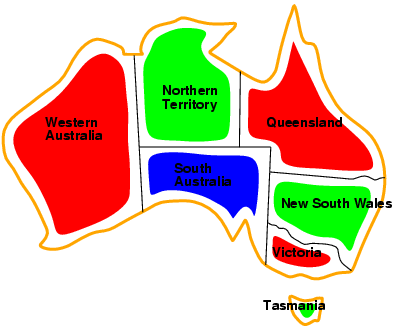What are the variables? What are the domains of values for each variable?

What are the constraints? No neighboring regions can have the same color. Can be expressed in a constraint graph with

• nodes representing variables
• edges representing pairwise (binary) constraints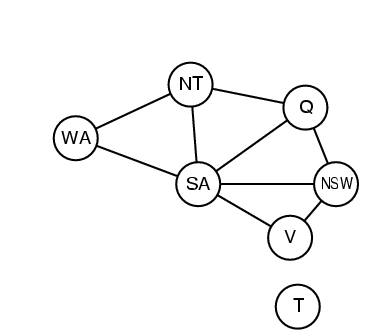A popular constraint propagation algorithm is AC-3. "AC" is for "arc-consistency". 3 is for third version. It proceeds by removing values from the domains of variables at either end of an arc to maintain the constraints.

A stronger version of this approach is called path-consistency (PC-2). It considers triplets of variables at a time.

WA NT SA Q NSW V T
1 (r,g,b) (r,g,b) (r,g,b) (r,g,b) (r,g,b) (r,g,b) (r,g,b)
2 WA-NT considering SA (r) (g) (b) (r,g,b) (r,g,b) (r,g,b) (r,g,b)
(g) (r) (b) (r,g,b) (r,g,b) (r,g,b) (r,g,b)
(b) (g) (r) (r,g,b) (r,g,b) (r,g,b) (r,g,b)
(b) (r) (g) (r,g,b) (r,g,b) (r,g,b) (r,g,b)
3 NT-SA considering Q (r) (g) (b) (r) (r,g,b) (r,g,b) (r,g,b)
(g) (r) (b) (g) (r,g,b) (r,g,b) (r,g,b)
(b) (g) (r) (b) (r,g,b) (r,g,b) (r,g,b)
(b) (r) (g) (b) (r,g,b) (r,g,b) (r,g,b)
4 and so on ...

Assign value to one variable at a time. Do depth-first search with backtracking.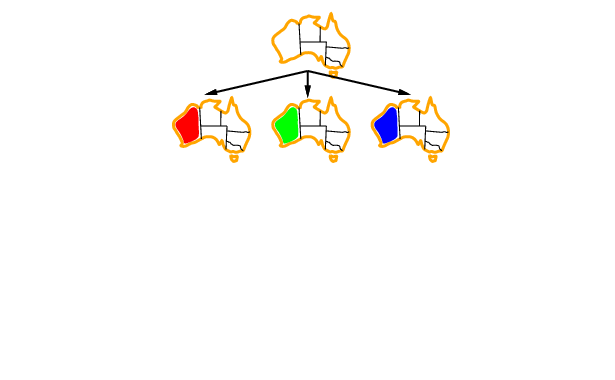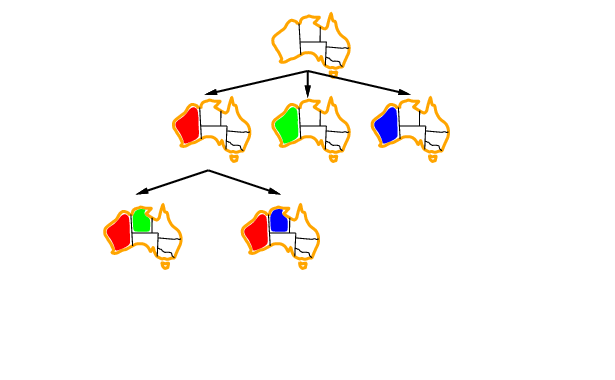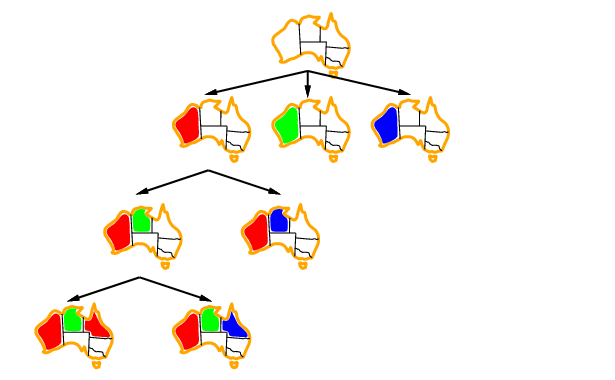Many ideas exist for guiding the depth-first search:

• selecting next variable to assign
• predefined order (not so good)
• MRV heuristic: variable with the minimum number of remaining values
• degree heuristic: variable with largest number of constraints with other unassigned variables
• selecting the next value to assign to the chosen variable
• least-constraining value heuristic: value that rules out the fewest remaining values in other variables
• propagate effect of an assignment, or inference
• forward checking heuristic: remove values from other variables that break constraints with the assignment just made,
• MAC heuristic: continue forward checking through other constraints affected by each new reduction in remaining values
• chronological backtracking: the usual depth-first backtracking, most recent
• backjumping: backtrack up further, to variable assignment that reduced the set of values available to the variable being assigned at the node where we fail

Here is the procedure, from Figure 6.8, in python and pseudo-code:

def min_conflicts(csp, max_steps):
assignment = dictionary indexed by variables with values randomly assigned
for i in range(max_steps):
if assignment is a solution for csp:
return assignment
conflicted_variables = set of variables involved in broken constraints
variable = one chosen randomly from conflicted_variables
value = value for variable with minimum number of conflicts
assignment[variable] = value
return 'failure'

## Applications of Min-Conflicts¶

### Map Coloring Problem¶

Let's try the map-coloring problem. What is the initial state? Must be a complete assignment. Let's just pick colors at random.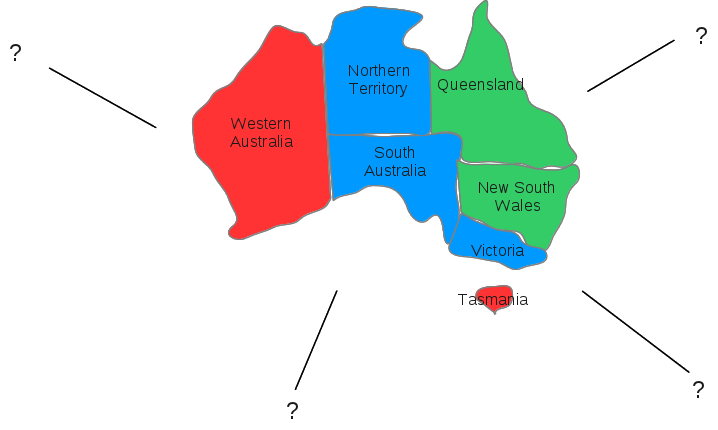Now what are the one-step neighbors of this starting state? Local search for CSP proceeds by randomly picking a variable from ones with assigned values that break a constraint. The new value assigned to that variable is one that has a minimum of conflicts with other variable assignments in this state.

Five variables have conflicts. Let's pick New South Wales, at random, among set of conflicted variables. Blue conflicts with two variables, red conflicts with none, and green conflicts with one. So, set color of New South Wales to red.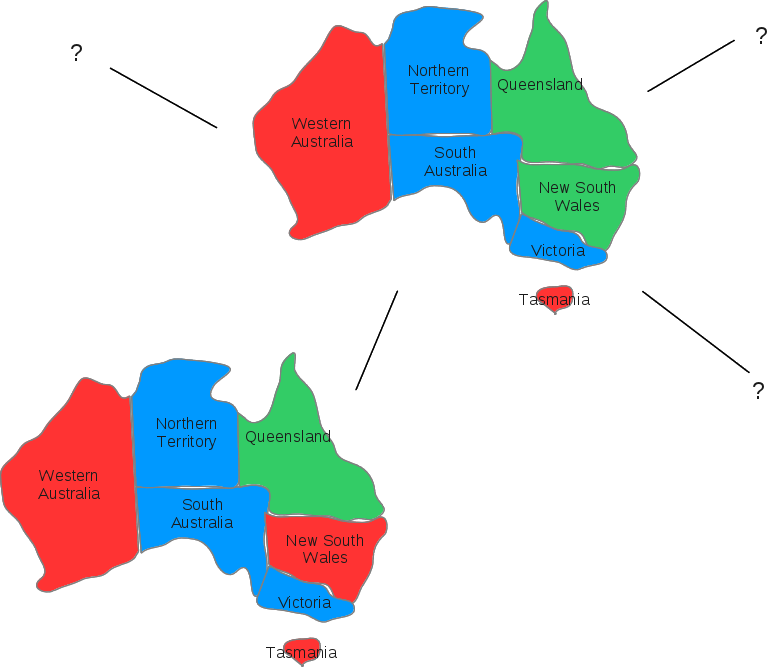Now three variables have conflicts. Let's pick Victoria. For Victoria, green has no conflicts, red and blue each have one, so pick green.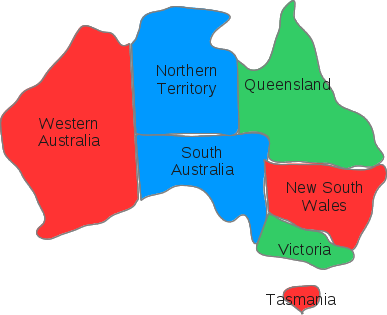Now two variables have conflicts. Pick Northern Territory. For Northern Territory, red, green and blue each conflict with one. Pick green randomly.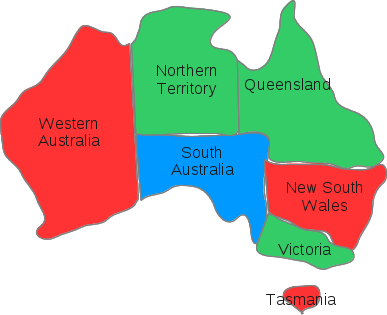Again, only two variables have conflicts. Pick Queensland. For Queensland, red, green and blue each have one conflict. Pick red randomly.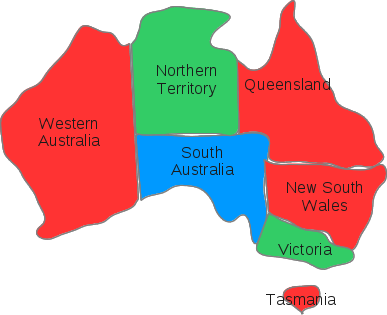Again, two variables. Pick New South Wales. For New South Wales, green, red and blue conflict with one. Pick green randomly.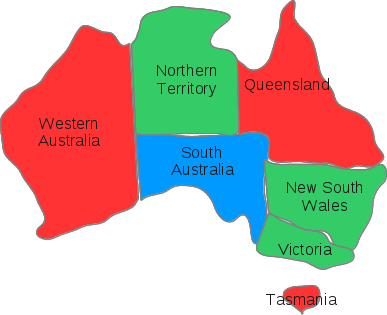Again, two variables. Pick Victoria. For Victoria, red has no conflicts, blue and green have one. So pick red.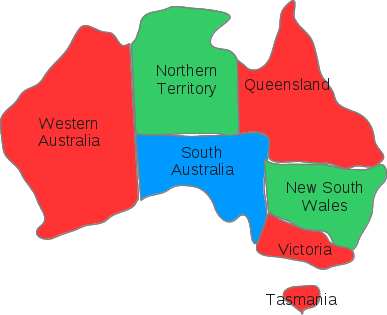And, TA-DA, no conflicts remain. We have found a solution.

### N-Queens Problem Solved¶

Place $n$ queens on a chess board such that no queen attacks another.

In figure below, $h$ is the number of pairs of queens that attack each other. We want to find an arrangement of queens for which $h=0$.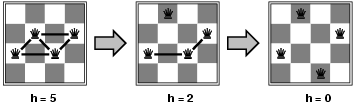Can solve 8-queens problem quickly, but for large $n$,$n$-queens problems take a very long time....right?

Wrong! Even when $n=1,000,000$ solutions can be found in an average of 50 steps!

Following figures are from Minton, et al.'s, paper linked below.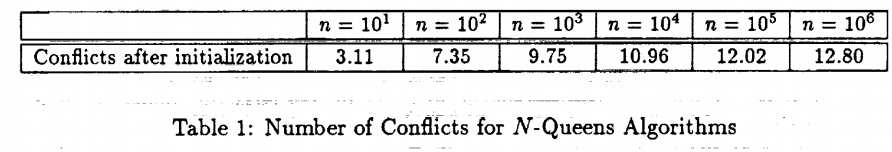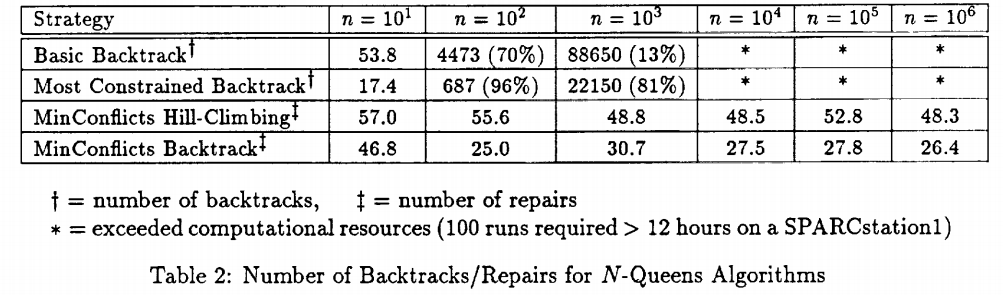Given a random initial state, min-conflicts can solve an n-queens problem in almost constant time for arbitrary $n$ with high probability (such as $n=10,000,000$).

A similar statement seems to be true for any randomly-generated CSP except in a narrow range of the ratio $R$: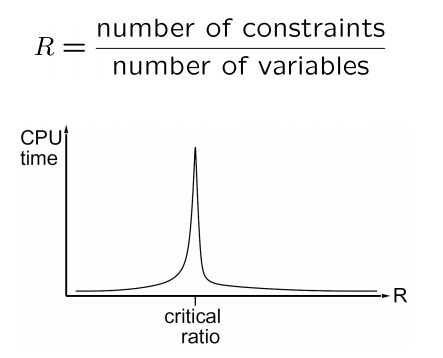### Scheduling Experiments on Hubble Space Telescope¶

Scheduling a week of tasks for the Hubble Space Telescope takes about 10 minutes with local search. Previously it had taken three weeks.from this site. And check out Hubble's amazing photos and videos.

The min-conflicts heuristic: Experimental and theoretical results, by Steven Minton, Andrew Philips, Mark Johnston, and Philip Laird, September 1991.

In [ ]: